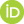# Skolem’s “paradox” as logic of ground: The mutual foundation of both proper and improper interpretations

Penchev, Vasil (2020) Skolem’s “paradox” as logic of ground: The mutual foundation of both proper and improper interpretations. [Preprint]Preview
Text
SkolemGround.pdf

## Abstract

A principle, according to which any scientific theory can be mathematized, is investigated. That theory is presupposed to be a consistent text, which can be exhaustedly represented by a certain mathematical structure constructively. In thus used, the term “theory” includes all hypotheses as yet unconfirmed as already rejected. The investigation of the sketch of a possible proof of the principle demonstrates that it should be accepted rather a metamathematical axiom about the relation of mathematics and reality.
Its investigation needs philosophical means. Husserl’s phenomenology is what is used, and then the conception of “bracketing reality” is modelled to generalize Peano arithmetic in its relation to set theory in the foundation of mathematics. The obtained model is equivalent to the generalization of Peano arithmetic by means of replacing the axiom of induction with that of transfinite induction.
A comparison to Mach’s doctrine is used to be revealed the fundamental and philosophical reductionism of Husserl’s phenomenology leading to a kind of Pythagoreanism in the final analysis.
Accepting or rejecting the principle, two kinds of mathematics appear differing from each other by its relation to reality. Accepting the principle, mathematics has to include reality within itself in a kind of Pythagoreanism. These two kinds are called in paper correspondingly Hilbert mathematics and Gödel mathematics. The sketch of the proof of the principle demonstrates that the generalization of Peano arithmetic as above can be interpreted as a model of Hilbert mathematics into Gödel mathematics therefore showing that the former is not less consistent than the latter, and the principle is an independent axiom.
An information interpretation of Hilbert mathematics is involved. It is a kind of ontology of information. Thus the problem which of the two mathematics is more relevant to our being (rather than reality for reality is external only to Gödel mathematics) is discussed. An information interpretation of the Schrödinger equation is involved to illustrate the above problem.

 Export/Citation: EndNote | BibTeX | Dublin Core | ASCII/Text Citation (Chicago) | HTML Citation | OpenURL
 Social Networking:

Item Type: Preprint
Creators:
CreatorsEmailORCIDPenchev, Vasilvasildinev@gmail.com
Keywords: axiom of choice; axiom of induction; axiom of transfinite induction; eidetic, phenomenological and transcendental reduction; epoché; Gödel mathematics; Hilbert mathematics; information; quantum mechanics, quantum information; phenomenology; principle of universal mathematizability
Subjects: Specific Sciences > Mathematics > Foundations
General Issues > Scientific Metaphysics
Depositing User: Prof. Vasil Penchev
Date Deposited: 17 Apr 2020 14:38
Item ID: 17082
Subjects: Specific Sciences > Mathematics > Foundations
General Issues > Scientific Metaphysics
Date: 16 April 2020
URI: http://philsci-archive.pitt.edu/id/eprint/17082

### Monthly Views for the past 3 yearsView Item